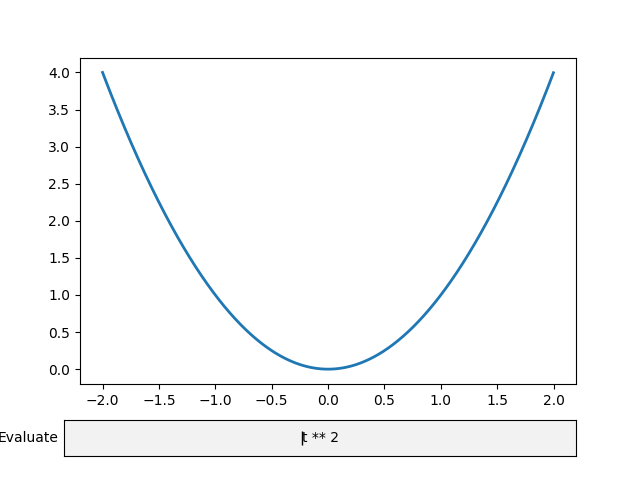# Textbox¶

The Textbox widget lets users interactively provide text input, including formulas. In this example, the plot is updated using the on_submit method. This method triggers the execution of the submit function when the user presses enter in the textbox or leaves the textbox.

Note: The matplotlib.widgets.TextBox widget is different from the following static elements: Annotations and Placing text boxes.

import numpy as np
import matplotlib.pyplot as plt
from matplotlib.widgets import TextBox

fig, ax = plt.subplots()

t = np.arange(-2.0, 2.0, 0.001)
l, = ax.plot(t, np.zeros_like(t), lw=2)

def submit(expression):
"""
Update the plotted function to the new math *expression*.

*expression* is a string using "t" as its independent variable, e.g.
"t ** 3".
"""
ydata = eval(expression)
l.set_ydata(ydata)
ax.relim()
ax.autoscale_view()
plt.draw()

axbox = fig.add_axes([0.1, 0.05, 0.8, 0.075])
text_box = TextBox(axbox, "Evaluate", textalignment="center")
text_box.on_submit(submit)
text_box.set_val("t ** 2")  # Trigger submit with the initial string.

plt.show()References

The use of the following functions, methods, classes and modules is shown in this example:

Keywords: matplotlib code example, codex, python plot, pyplot Gallery generated by Sphinx-Gallery